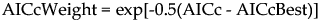Fitting Linear Models > Stepwise Regression Models > The Model Averaging Option
Publication date: 08/13/2020

# The Model Averaging Option

The model averaging technique enables you to average the fits for several models, instead of selecting a single model. Often, the resulting average model has better prediction capability than each of the single models that were averaged. The Model Averaging feature is useful for avoiding a model that over fits your data. When many terms are selected into a model, the fit tends to inflate the parameter estimates. Model averaging tends to shrink the estimates on the weaker terms, which yields better predictions. The models are averaged with respect to the AICc Weight of each model, which is calculated as follows:AICcBest is the smallest AICc value among the fitted models. The AICc Weight values are calculated for each model, sorted in decreasing order, and scaled to sum to 1. The scaled AICc Weight values that sum to less than one minus the specified Cumulative AICc Weight Cutoff value are set to zero. This eliminates the use of weak models in the averaged model. The parameters for the averaged model are the weighted averages of the parameter estimates across the models that have nonzero AICcWeights.